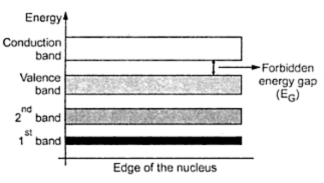### The energy Band Theory

We have seen that every shell is associated with an energy level. An electron orbiting very close to the nucleus in the first shell is very much tightly bound to the nucleus and possesses only a small amount of energy. Hence first shell has lowest energy level. Greater the distance of an electron from the nucleus, the greater is its energy. Hence the energy level of the outermost shell is highest. Due to such high energy, the valence electrons in the outermost shell can be easily extracted out and hence such electrons take part in chemical reactions and in bonding the atoms together. Now this discussion is related to the electrons and shells of one isolated atom only.
In solids, atoms are brought close together. In such a case, outer shell electrons are shared by more than one atom. So these electrons come under the influence of forces from other atoms too. The valence electrons are shared by forming a bond with the valence electrons of an adjacent atom. Such bonds are called covalent bonds. Thus the valence electrons are not free under normal conditions, as they are shared by the adjacent atoms.
Now the valence electrons possess highest energy level. When such electrons from the covalent bonds, due to the coupling between the valence electrons, the energy levels associated with the valence electrons merge into each other. This merging forms an energy band.
Similarly the energy levels of various electrons present in the first orbit, second orbit etc. also merge to form the various energy bands.
So instead of the presence of widely separated energy levels as that of the isolated atoms, the closely spaced energy levels are present in a solid, which are called energy bands.
Out of all the energy bands, three bands are most important to understand the behaviour of solids. These bands are,
1) Valence band,  2) Conduction band,  3) Forbidden band or gap
Key Point : The energy band formed due to merging of energy levels associated with the valence electrons i.e. electrons in the last shell, is called valence band.
As mentioned earlier in normal condition, valence electrons form the covalent bonds and are not free. But when certain energy is imparted to them, they become free.
Key Point : The energy band formed due to merging of energy levels associated with the free electrons is called conduction band.
Under normal condition, the conduction band is empty and once energy is imparted, the valence electrons jump from valence band to conduction band and become free.
While jumping from valence band to conduction band, the electrons have to cross an energy gap.
Key Point : The energy gap which is present separating the conduction band and the valence band is called forbidden band or forbidden gap.
The energy imparted to the electrons must be greater than the electrons must be greater than the energy associated with the forbidden gap, to extract the electrons from valence band and transfer them to conduction band. The energy associated with forbidden band is denoted as EG.
Key Point : The electrons can not exist in the forbidden gap.
The graphical representation of the energy bands in a solid is called energy band diagram. Such an energy band diagram for a solid silicon is shown in the Fig. 1.Fig. 1 Energy band diagram
The electrons in the various orbits revolving around the nucleus occupy the various band including fully or partly occupied valence band. The conduction band which is normally empty carries the electrons which get drifted from the valence band. These electrons present in the conduction band are free electrons and they drift about in the spaces between the atoms.
For any given type of material the forbidden energy gap may be large, small or nonexistent. The classification of materials as insulators, conductors or semiconductors is mainly dependent on the widths of the forbidden energy gap. Let us see the classification of materials as insulators, conductors and semiconductors based on energy band diagram. Before that let us see the unit in which energy associated with the bands is measured.
1.1 The eV, Unit of Energy
The energy is measured in joules (J) in the M.K.S. system. As mentioned earlier, each electrons has an energy level associated with it. The unit joule is very large for the discussion of such energies associated with electrons. Hence such energies associated with the electrons are measured in electrons are measured in electrons volts denoted as eV.
The charge on a single electron is 1.6 x 10-19 coulomb. So one electron volt is defined as the energy required by an electron to fall through a potential of one volt.
...      1 eV = 1.6 x 10-19   (C) x 1 (V) = 1.6 x 10-19 J